When working with Calculated Items, you can use Excel Formulas right away (as long as you do not reference any external cells) such as SUM, IF, OR, AND, AVERAGE, etc.

Here is our current Pivot Table setup: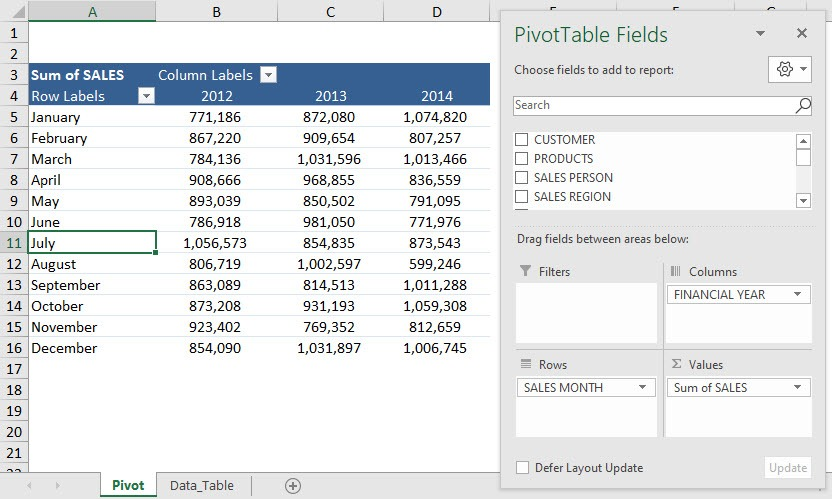You can easily add another row that calculates the average sales for each year using formulas in calculated items. Let’s see how it can be done!

Download the Excel Workbook below and follow the step-by-step tutorial below to add Excel formulas in Calculated Items.

STEP 1: Click on any cell in the Pivot Table.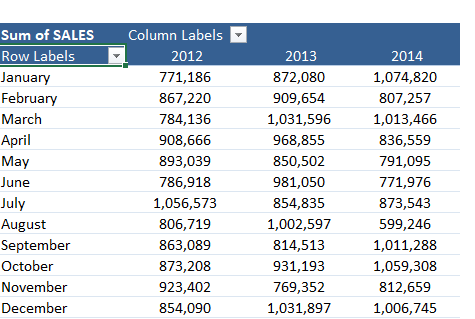This will display PivotTable Analyze and Design tabs.

STEP 2: Go to PivotTable Analyze > Fields, Items & Sets > Calculated Item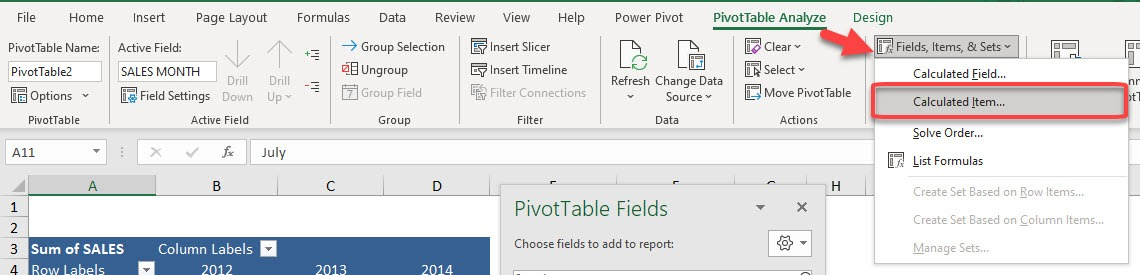STEP 3: In the Name box, type in a name that you prefer for the formula.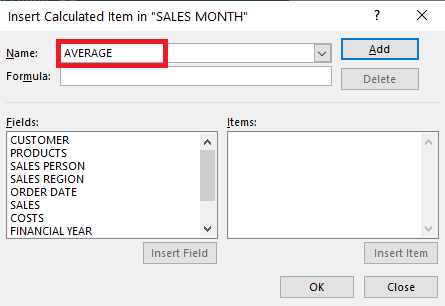STEP 4: In the Formula box, type in the Average Function:

= AVERAGE(January,February,March,April,May,June,July,August,September,October,November,December)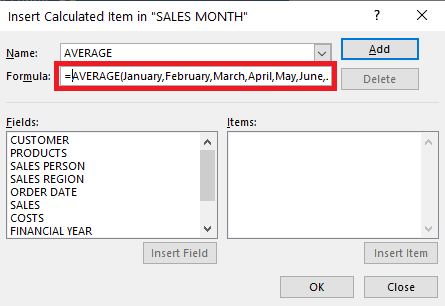STEP 5: Click Add then OK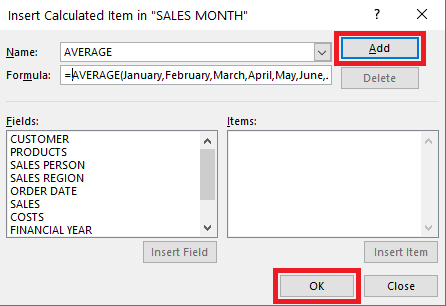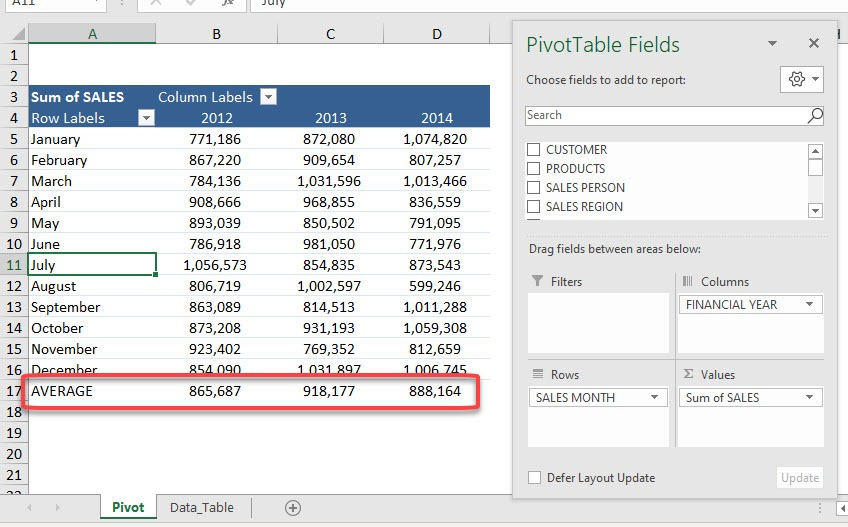Edit Formula in Calculated Item

If you wish to edit the formula from an average of 12 months to an average of 3 months, you can do that as well:

STEP 1: Click on any cell in the Pivot Table.This will display PivotTable Analyze and Design tabs.

STEP 2: Go to PivotTable Analyze > Fields, Items & Sets > Calculated ItemSTEP 3: In the Name box, select the formula.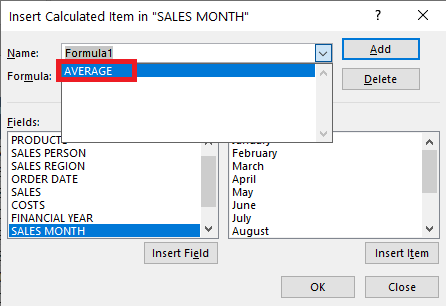STEP 4: Edit the formula.

= AVERAGE(January,February,March)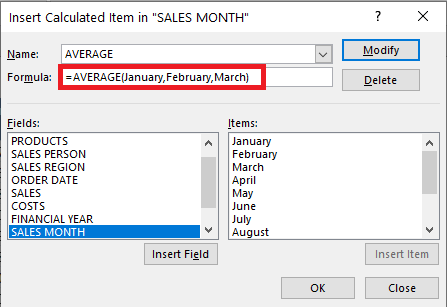STEP 5: Click Modify.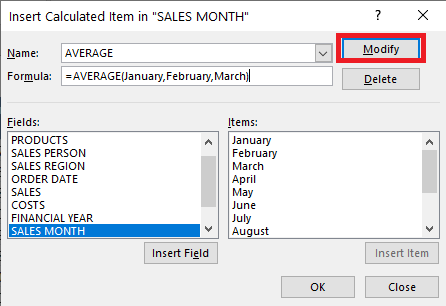STEP 6: Click OK.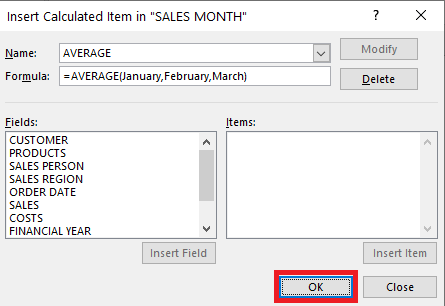Delete Formula in Calculated Item

You can delete the formula from the Pivot Table calculated item by following the steps below:

STEP 1: Click on any cell in the Pivot Table.STEP 2: Go to PivotTable Analyze > Fields, Items & Sets > Calculated ItemSTEP 3: In the Name box, select the formula.STEP 4: Click Delete.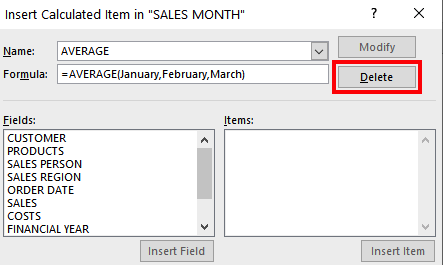This is how you can easily create formulas in a calculated item, edit them or even delete them if it is not required anymore.